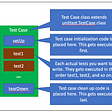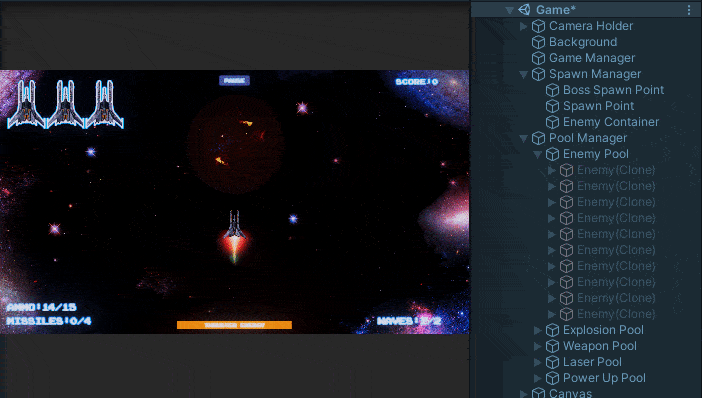# What is Quick Sort

Quicksort is an algorithm that sorts an array of n elements in O(n²) time in the worst case. In this worst case, quicksort is slow, but the average execution time is O(n log n), which is very efficient, and the constant factor hidden in O(n log n) is very small.

Like merge sort, quicksort is based on divide-and-conquer. Therefore, the operation of the quick sort can be described as follows.

## Split

Split the array A[p..r] into two subarrays A[p..q-1] and A[q + 1..r]. Put an element less than or equal to q into A[p..q-1] and an element greater than or equal to q into A[q + 1..r]. We compute the index q for this partitioning process.

## Conquer

A recursive call to quicksort sorts the two subarrays A[p..q-1] and A[q + 1..r].

## Concatenation

This operation is not necessary because the subarray is already sorted.

The following code implements quick sort.

`def Quicksort(A, p, r): if p < r:  q = Partition(A, p, r)  Quicksort(A, p, q - 1)  Quicksort(A, q + 1, r)`
1. Make sure the array is large enough.
2. Find the index q and sort small values to the left and large values to the right according to the size of q.
3. Quicksort is called recursively.

The following is the code of Partition

`x = A[r]i = p - 1for j = p to r - 1: if A[j] <= x:  i = i + 1  A[i]와 A[j] 교환A[i + 1]와 A[r] 교환return i + 1`

# Conclusion

Quicksort is a sorting algorithm using the divide-and-conquer method. In the worst case, it takes O(n²) time, but on average it is a very efficient algorithm that ends in O(n log n) time. Even as I will explain later, in most cases, it can be seen that it ends in O(n log n) time very efficiently. In the next post, we will look at the performance of quicksort.

--

--

--

## More from ki hyun Lee

Full Stack Dev

Love podcasts or audiobooks? Learn on the go with our new app.

## A Quick Start Guide to Python unittest (Part 1)## Feature: Enemy Shields## Three Techniques That Will Improve Your Coding Skills## An Introduction to Python Matplotlib with 40 Basic Examples## CS 373 Spring 2021 Blog 12: Ian Trowbridge## Understand Your System Behavior By Implement Distributed Tracing Using Jaeger## Importance of Selecting Right Automation Testing Tool## Space Shooter Refactoring : Pooling System— Part 1Full Stack Dev

## Summer Term Applications Will Open Soon## Docker !! [ with practical of course ; )]## Netflix: SMART Goals and KPI’s